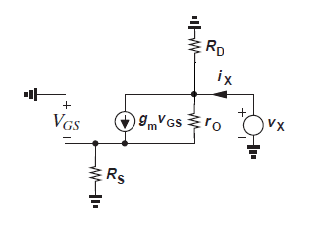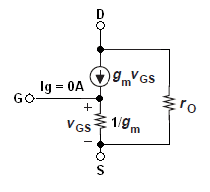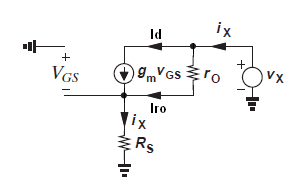# FET common source analysis with source resistor

naman chauhan
Hi everyone,I have attached an image of fet amplifier in common source configuration. I want to have the ac analysis of this circuit. I am able to figure out the input impedance and the voltage gain. But I can't formulate for output impedance.

According to me, while calculating output impedance input should be zero. As soon as vs becomes zero the value of current (gm Vgs) will be zero. So the current source will behave as an open circuit. And we get (Rd||rd). But according to book it's wrong.

Can any body help!

naman chauhan
Can you tell me what is a rd resistance. rd should not be connected between drain and source (parallel to the gm*Vgs current source) ?

well rd is the resistanace offered by FET. Its the internal resistance of the device. It is inverse of the trannsconductance offered by FET.
rd= 1/gm

Jony130
well rd is the resistanace offered by FET. Its the internal resistance of the device. It is inverse of the trannsconductance offered by FET.
rd= 1/gm
No, that completely wrong. rd = ro = ΔVds/ΔId at constant Vgs - is a small-signal internal resistance parallel to the gm*Vgs current source. But not equal to 1/gm.
So you need to use this small-signal model to find output impedance.Zo = Vx/Ix

#### Attachments

naman chauhan
as far as my understanding says, gm= Id/Vgs and if the slope of characterstic curve is constant the gm can be approximated as rd. Please correct me if i am worng.

In the image attached by you the current source should be open because at the time of calculating output impedence we remove input voltage source. since Vgs=0, current source is open circuit. so if we remove the current source then the output impedece will be, Zo=(ro+Rs)||Rd.
Is this the way to do it?

Jony130
as far as my understanding says, gm= Id/Vgs and if the slope of characterstic curve is constant the gm can be approximated as rd. Please correct me if i am worng.
Yes, you are absolutely right. But if you want to use this 1/gm resistance in your small-signal model. You need to change your model to this one:And now the voltage gain is equal to

Av = Rd/(1/gm + Rs)

Also notice that ro = rds = ΔVds/ΔId represent channel-length modulation similar to the Early effect in bipolar devices. And to represent channel-length modulation, i.e., variation of Id with Vds we add a resistor ro to small signal model.

In the image attached by you the current source should be open because at the time of calculating output impedence we remove input voltage source. since Vgs=0, current source is open circuit. so if we remove the current source then the output impedece will be, Zo=(ro+Rs)||Rd.
Is this the way to do it?
Yes is is true that when we calculating output impedance we short a input voltage source.
So we connect the FET gate to ground. But we also replace the load resistance with a Vx test voltage source. So we can find Zo = Vx/Ix

So if you look at the circuit diagramYou will see that Vgs is not equal 0V (only gate is connect to GND) not the source.
Vgs = -Is * Rs
Test voltage provide a current for Rs resistor so there will be a voltage drop across Rs resistor, so the gm*Vgs current source is not a open circuit. There will be additional current flow through Rs resistor. Is = Iro + (gm*Vgs).

But if we remove ro resistance from our small-signal model, then Vgs is indeed equal to Vgs = 0V, current source is open circuit. The output impedance will be, Zo = Rd.

#### Attachments

Last edited:
naman chauhan
thanks a lot for telling me the difference in between gm and rd. I had a missconfussion regarding this concept. thanks for sharing this with me.

so Vo=Vds+Vs
where, Vds = (Io-gmVgs)*(ro||Rd): here Vgs=-Vs
Vds=(Io+gmVs)*(ro||Rd)

Vs=Rs*(Io) sum of current source and ro current will be the source current.

Vo=Vds+Vs

Vo=(Io+gmVs)*(ro||Rd)+(Rs*Io)
or
Vo=((Io+gm(Rs*Io))*(ro||Rd))+(Rs*Io)
Vo=Io*((1+gmRs))*(ro||Rd))+(Rs)
Vo/Io=((1+gmRs))*(ro||Rd))+(Rs)

right...?

but text books says the zo=Rd/(1+gmRs).

Jony130
Try to find Zo if you remove Rd from the circuit.Vx - Iro•ro - Ix•Rs = 0 (1)

Ix = gm•Vgs + Iro (2)

Vgs = (-Ix)•Rs (3)

And now I substituting equation (2) and (3) to (1)

Vx - Iro•ro - (gm•(-Ix) •Rs + Iro)•Rs = 0

Now solve for Iro

Iro = (gm• Ix• Rs² + Vx) / (Rs + ro)
(4)

And substituting (4) to (1) and solve for Ix

Ix = Vx / (Rs + ro + gm•Rs•ro)

And finally

Vx/Ix = = Re + ro + gm•Re•ro = ro • (1+gm•RE + RE/ro)

Or we can write this equation

Ix•Rs + Iro•ro = Vx (1)

Iro = (Ix - Id) (2)

Id = (gm•(-Ix)•Rs) (3)

so after substitution

Ix•Rs + ( Ix - (gm•(-Ix)•Rs))*ro = Vx

next solve for I2

Ix = Vx / ( Rs + ro + gm*Rs*ro )

Vx/Ix = Rs + ro + gm*Rs*ro

so now if we add Rd resistor we have this 'exact' value

Rout = Rd||(ro+Rs+gm•ro•Rs) = Rd||( ro •(1+gm•Rs + Rs/ro) )

This expression, Rout = RD || (ro * (1 + gm * Rs)), is a good approximation provided that ro >> 1/gm.

Can you tell me the title of the book?

#### Attachments

Last edited:
naman chauhan
Hey thanks a lot. I get it where I was wrong. I thought that ro is directly connected to ground but it's connected to Rs. That's why I am taking Rd||ro.
Thanks a lot again.